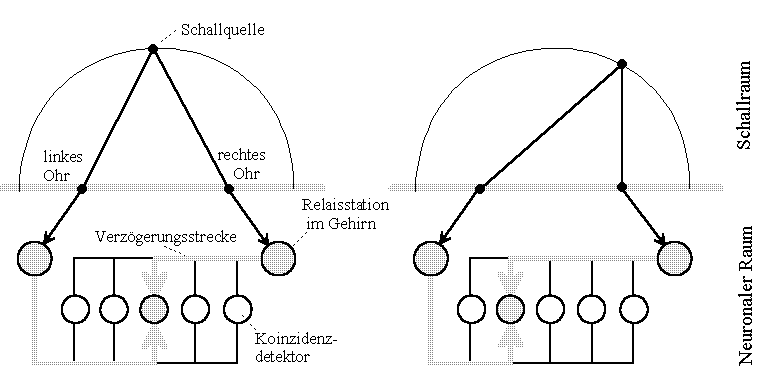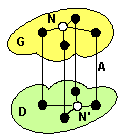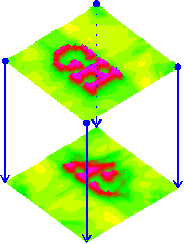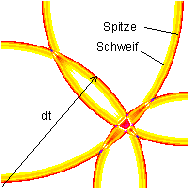# Projections and Interference Pattern in Nerve Networks

G. Heinz

### History

Following Konishi [1a] Lloyd A. Jeffress 1948 at Caltech [1b] had a simple idea to understand the principle of localisation of sound sources in space, Fig.1. These fundamental circuit, today we would call it an 'inter-medial interference circuit', shows important features of biological informatics in a new sight: a correspondence between a location and the transmitting time-functions, a connection between code in space and time. (Jeffress published only a different drawing, Fig.2).Fig.1: Konishi's drawing of Jeffress idea 1993 [1a]Fig.2: Original circuit of Jeffress paper 1947 [1b] - historical the first inter-medial interference circuit

The circuit Fig.1 has interesting properties:

• Time-functions (impulses) expand relative to the other
• A wire is not a potential, it has delaying properties and distributed parameters
• Space and projection appears as a mirrored projection with the proportion dx/-dx' = v dt/ -v' dt
• space function (length dx) and time function (lenght dt) of an impulse are combined with ds = v dx
• The output of a detecting element is dependent of the lenght and velocity of input wires (!)
• Logical elements (detectors) have non-deterministic behaviour
• Instead of a neural type the location of a neurone is connected to the logical function of a neuron (!!!)
• Data addressing is possible in short-connected networks (!) and
• It is necessary for data addressing, to have short connected networks (!)
• The cover of a nerve web, not the connectivity, codes functional properties (!!!)
• The logical signal flow is not represented by wiring, but by relative delays, that are dependent of covers, velocities and locations
• Stochastic, non-deterministic wiring is possible without general short-cut

Also if Konishi discussed periodical time functions: interference circuits works as better, as more the time functions have non-sinoidal character. Nerve pulses have velocities from µm/d...m/s, time-length in the range 0.1 µs...s and wave length in the range µm...cm.

A specific detail seems that the circuit don't need different media to work. It also works between two neural fields connected by some axons.

To answer te question, why nobody has found these interesting properties over 50 years, we have to remember the history of computations to the 40's. Behind Conrad Zuse (Z1 in 1938) lots of scientists tried to develope first computers. In the beginning forties the fiction of machinery computation moved the minds. Discretisation of analog signals should be the way, to make things going in a digitized world. Electrical wires imploded to potentials, to nodes.

In the beginning fifties such 'neural' nets were qualified to learn. Delay models of wires seem to be unnecessary (Perceptron, backpropagation etc.) to understand neural networks. Perhaps Jeffress model was not of interest. Even some biologists as Konishi remembered him all times to interpret acoustical experiments.

The genius of computing and neural pioneers was, to digitize Jeffress interference circuit. A time-function was interpreted in discrete values, delay portions were called states or samples. State machines, Petri-nets and digital filters were born.

While McCulloch/Pitts started the paper  in 1943 with the words

"The velocity along the axon varies directly with its diameter, from less than one meter per second in thin axons, which are usually short, to more than 150 meters per second in thick axons..."

they expressed neural circuits with axons and dendrites in the same minute with state sequences instead of time functions.
What a mistakes with fatal consequences!
In the physical assumptions  we read:

"...
2. A certain fixed number of synapses must be excited within the period of latent addition in order to excite a neurone at any time, and this number is independent of previous activity and position on the neurone.
3. The only significant delay within the nervous system is synaptic delay.
..."

What a mistake! It is not possible, to find any delaying time-function in the paper. Else we find terms, characterising the new age, the age of computer technology. Instead of terms in form of delaying time-functions like

f1(t) = f2(t-T1) + f3(t-T2) + ...

where T1 and T2 are (float number-) delays of real wires proportional to distances, by analogy we find integer terms of the form

N3(t) :=: .N1(t-1) .v .N2(t-3)

The numbers 1, 3 mark time-steps, the "v" marks, what we call today a logical OR (please excuse the simplified way to describe the formulas under HTML).

The way to describe delays as integer numbers dominated the neurocomputing science (Neural Networks) for 49 years . So all the time it was not able, to find any neural projection, because pulse waves were unknown.

Why I found out this fact in 1992? Only because I thought about slow conduction speeds of nerve pulses in dendrites: animals have to be fast to get a chance in evolution. Whats the reason, that impulses flow so slowly?

So, in one of the origins of neuro-computing we find a much simplified description of physical reality. In sight to the development of computers (state machines) this might be a very helpful way. But it covered ideas in the direction to interference, wave fields, relativity of time. The meaning of an intrinsic delay of a neural wire was lost for years. A time function in the form f(t-T) was replaced by a state- or sample sequence N(i), N(i+1), N(i+2)... .

Also forthcoming models did not note incremental delays of wires. The 'synapse' was later recognized as the learning weight of a neuron. So papers, discussing the phenomenon of non-interpretability of bio-neural networks  with "neural networks" were common sense.

In this sight, Jeffress imagination was a short lightning flash in the dark.

At the other hand, the models of Nobel-price candidates Hodgkin/Huxley (1952)  were precise enough to discover interferential projections. But, be it, as it is, these models are over-loaded, they are to complex to recognize interference projections. They are to slow, to simulate some thousands of neurones in a time analysis manner within years.

Today it is time to recognize, that quantisation had produced this mistakes. At the one hand, PSI-simulations show , time and space properties have the very impact on the attainability of interference projections. At the other hand, the re-interpretation of biological networks only is possible in case of structural equivalence. The real structure of a neural net can be interpreted better with delays and time-functions then by state machines. We have forgotten, that a state sequence is a special, discrete form of a time-function. At last, time functions develope themselves physical properties characterizing delaying spaces. So a simple divergence of a wire that's ends converge diametric produces Youngs or Huygens double split pattern.

I did not know Jeffress or any interference system, when I realized theoretical an electrical interference circuit in optical analogy in 1992. It had the property, to produce only mirrored projections.

In opposite to other works, calculations in this homepage are only produced using time functions and free wave propagation. Following, it don't exist a potential of a wire. Instead, we have to calculate wires with distributed parameters, potentials are connected to locations (x,y,z) in space. Moves a time-function through space, the delay term T will increase proportional to the distance from the point of excitement.

A wave moving through space therefore is interpreted as a circular wave, also, if this may be a simplification for the interpretation of neural systems.

### Pictures of Thought

From nervous system we know topological maps and mirrored projections. The terminus 'projections' is used since years, although we didn't understand the reason in detail.
Comparable to mirrored projection of optical delay spaces, projections are possible between non-deterministic connected networks. Therefore we have to simulate very careful the waves, while pulses move at all possible ways through the network.
Should biological systems partially work as interference systems, very special measuring methods are able to interpret them. PSI-Tools was developed, to calculate excitement maps from channel data. With some luck, we hope to be able to interpret first (measured) pictures of thought. That means, we try to calculate excitement maps from nerve net signals. The first simulation of a pulse-projection, transfered over three channels (axons), we got in november 1994 - it is the dark background image of the homepage.

### What is an Interference (or Superimposing) Circuit?

The puls-like character of nerve signal flow offers, that boolean methods are not able to interpret informational tasks of a nerve nets. Any signal processing task in a non-deterministic wired network can only occur on a place, where different pulses meet each other. Thus logical processing appears localisation sensitive. Better: relations in space code the function of the net! May be, this is a very new idea with consequences.
To understand what this means, a simple circuit is used.

The most simple interference circuit can be built with an two input operating element (multiplier, adder...) that's output drives one input. Also a two input element, connected with both inputs to one node via different delays can be such an interference circuit. In my book (N.I., 1993), I discussed different simple interference circuits, showing very interesting properties in space-, time- and frequency domain.

A more general form we get by connecting two fields (a generating G and a detecting D) together with some axons (A). We get a structure, that may be known from anatomical drawings. Every neurone (N, N') of both fields has a connection to every end-point of the connecting axons, whereby all wires have length-proportional delays.Viewing any impulse, outgoing from a generator neuron (G) in all directions, we remark, that all partial impulses under circumstances can meet each other on the detecting field (D) in the place, where delays over all possible ways are the same.
These simple interference circuit produces mirrored projections and images (whenever) between generating- (G) and detecting space (D). Projections of thought.

### How to simulate interference networks?

PSI-Tools (Parallel and Serial Interference Tools) allows to calculate simple interference circuits, consisting of generator and detector fields in space. Neural fields considered as dense connected. Delays may be proportional to distances and velocities (homogenous space).

It is able, to solve the following tasks:

• Recording of parallel channel data (16 channels, 50 kSamples/s)
• Synthesis of channel data from a virtual generator space (drawn bitmaps)
• Processing of channel data (filtering, offset compensation, appending channel data streams...)
• Calculation of interference integrals in area or in space (excitement maps), interference movies (wave fields), interference classes analysis, electrode maps etc.

Resulting excitement maps are calculable on different ways: as interference integrals or as wave fields. We calculate interference integrals point by point and movies time step by time step, but with the same algorithm, the authors H-interference transformation (HIT).
Co-ordinates of each channel source or sink can be choosen freely. Generator and detector fields can have different velocities, origins, orientations and sizes. They are designed as bitmaps with variable and independent matrix- and physical dimensions. Timing, sample rate, pulses form and intervall, pulse distances can be varied.
The help-file of PSI-Tools is downloadable from the references chapter on this homepage.

### Reconstruction contra Projection

Excitement maps are interesting in two directions: first, technical tasks demand reconstruction of the original space, of the generator space. Second, we are interested to calculate projections onto other spaces to simulate neural fields.
A projection is mathematical dual to a reconstruction on the same field through an inversed time function. Default is the reconstruction with growing time values. To produce a projection, one has to revert the channel data.Fig.: Reconstruction (top) and projection (bottom) as interference integral from the same, synthesized channel data stream. Both fields have identical properties, velocity is the same. Channels have the same delay. Because of over-conditioning (one channel to much) we find high projection quality only in the center range.

We can recognize two conditions for projections: 1) geometrical impulse length have to be smaller then structures, 2) small channel numbers supposed, the pause between following impulses has to be large against the (time domain) field size. Time- and space-units are combined via medial velocity.

### Time Sequence Maps contra Projections

Behind image-like elements channel data also carry sensoric amplitudes. Thus the result of an interference analysis are non-avoidable projective elements combined with interference pattern. Following, the interference transformation calculates a map of interference pattern (sound- or noise-picture) in the same way, as a projection or reconstruction. The interferencial pattern component can be seen, when pulses reach the detector field in fast rates. This can be a consequence of very small pausing intervals of transmitting axons. Then a wave comes in interference not only with itself, together a wave interferes with following and preceding waves. Consequently, the interference integral appears mapped into interference pattern, independent, if we calculate a reconstruction or projection. In case, subsequent impulses follows in shortest sequence, the total detector field will come into interference, projections disappear. May be, this could be an interpretation of pain from physical point of view.Fig.: Part of an four channel interference movie (PSI-Tools): snap shot of four waves, moving over the detecting field. The channel source points are located in the corners of the field. We observe the time step short after a four-times interference.

### Conclusion

The team has since 1994 the chance to inspect simulated and recorded interference projections. It is possible, to simulate, to analyse or to develop simple interference circuits. Also it is possible, to record or synthesize channel data. PSI-Tools can reconstruct excitement maps of the exciting space even, as PSI-Tools can calculate projections in a second space. Projections appear mirrored or holographic, while reconstructions appear non-mirrored.
Behind neural applications PSI-Tools is useful, to reconstruct EEG-, ECoG-, EKG- or US-data streams. It is possible, to compute interference integrals as excitement maps or as wave field movies.

### References

[1a] Konishi, M.: Die Schallortung der Schleiereule. Spektrum der Wissenschaft, Juni 1993, S. 58 ff.

[1b] Jeffress, L.A.: A place theory of sound localization. Journ. Comparative Physiol. Psychol., 41, (1948), pp.35–39

 McCulloch, W.S.; Pitts, W.: A logical calculus of the ideas immanent in nervous activity. Bulletin of Mathematical Biophysics 5:115-133

 Widrow, B., Hoff, M.E. Adaptive switching circuits. 1960 IRE WESCON Convention Record, New York: IRE, pp. 96-104

 Rumelhart, D.E., McClelland, J.L.: A Distributed Model of Human Learning and Memory. in: Parallel Distributed Processing. Bradford/MIT Press Cambridge, Massachusetts, vol. 2, eighth printing 1988.

 Hodgkin, A.L., Huxley, A.F.: A Quantitative Description of Membrane Current and Its Application to Conduction and Excitation in Nerve. Journ. Physiology, London, 117 (1952) pp. 500-544

 About the thumb-experiment see IWK Ilmenau, 1994 (german)

Page-URL: historic/intro/intro.htm